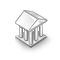Interesting script?
So post a link to it -
let others appraise it

You liked the script? Try it in the MetaTrader 5 terminal# IncColors - library for MetaTrader 5

Views:
3278
Rating:
Published:
2012.05.14 16:07
Updated:
2016.11.22 07:32

This class contains functions for working with colors. It has color conversion and other useful functions.

Conversion RGB to HSV

`void RGBtoHSV(double aR,double aG,double aB,double & oH,double & oS,double & oV)`

Functions from http://www.easyrgb.com/index.php?X=MATH. All these function have 6 arguments: first 3 are parameters, the next 3 are conversion results, passed by reference.

Conversion RGB to XYZ

`void RGBtoXYZ(double aR,double aG,double aB,double & oX,double & oY,double & oZ)`
Conversion XYZ to RGB
`void XYZtoRGB(double aX,double aY,double aZ,double & oR,double & oG,double & oB)`
Conversion XYZ to Yxy
`void XYZtoYxy(double aX,double aY,double aZ,double & oY,double & ox,double & oy)`

Conversion Yxy to XYZ

`void XYZtoYxy(double aX,double aY,double aZ,double & oY,double & ox,double & oy)`
Conversion XYZ to HunterLab
`void XYZtoHunterLab(double aX,double aY,double aZ,double & oL,double & oa,double & ob)`
Conversion HunterLab to XYZ
`void HunterLabToXYZ(double aL,double aa,double ab,double & oX,double & oY,double & oZ)`
Conversion XYZ to CIELab
`void XYZtoCIELab(double aX,double aY,double aZ,double & oCIEL,double & oCIEa,double & oCIEb)`
Conversion CIELab to ToXYZ
`void CIELabToXYZ(double aCIEL,double aCIEa,double aCIEb,double & oX,double & oY,double & oZ)`
Conversion CIELab to CIELCH
`void CIELabToCIELCH(double aCIEL,double aCIEa,double aCIEb,double & oCIEL,double & oCIEC,double & oCIEH)`

Conversion CIELCH to CIELab

`void CIELCHtoCIELab(double aCIEL,double aCIEC,double aCIEH,double & oCIEL,double & oCIEa,double & oCIEb)`

Conversion XYZ to CIELuv

`void XYZtoCIELuv(double aX,double aY,double aZ,double & oCIEL,double & oCIEu,double & oCIEv)`
Conversion CIELuv to XYZ
`void CIELuvToXYZ(double aCIEL,double aCIEu,double aCIEv,double & oX,double & oY,double & oZ)`
Conversion RGB to HSL
`void RGBtoHSL(double aR,double aG,double aB,double & oH,double & oS,double & oL)`
Conversion HSL to RGB
`void HSLtoRGB(double aH,double aS,double aL,double & oR,double & oG,double & oB)`

Conversion RGB to HSV

`void RGBtoHSV(double aR,double aG,double aB,double & oH,double & oS,double & oV) `
Conversion HSV to RGB
`void HSVtoRGB(double aH,double aS,double aV,double & oR,double & oG,double & oB)`
Conversion RGB to CMY
`void RGBtoCMY(double aR,double aG,double aB,double & oC,double & oM,double & oY)`
Conversion CMY to RGB
`void CMYtoRGB(double aC,double aM,double aY,double & oR,double & oG,double & oB)`
Conversion CMY to CMYK
`void CMYtoCMYK(double aC,double aM,double aY,double & oC,double & oM,double & oY,double & oK)`
Conversion CMYK to CMY
`void CMYKtoCMY(double aC,double aM,double aY,double aK,double & oC,double & oM,double & oY)`

Other functions

Get RGB components

First parameter - color, the next arguments are color components, passed by reference.

`void ColorToRGB(color aColor,double & aR,double & aG,double & aB)`
Get R component
`double GetR(color aColor)`
Get G component
`double GetG(color aColor)`
Get B component
`double GetB(color aColor)`

Conversion RGB to color

`color RGBToColor(double aR,double aG,double aB)`
Get mixed color between 2 colors
`color MixColors(color aCol1,color aCol2,double aK) // aK - from 0 to 1`
```void Gradient( color & aColors[], // list of colors
color & aOut[],                  // output array
int aOutCount,                     // Size of output array
bool aCycle=false                  // cyclic gradient (the out array ends with starting color)
)```
Another simple conversion RGB -> XYZ and its inverse XYZ->RGB
```void RGBtoXYZsimple(double aR,double aG,double aB,double & oX,double & oY,double & oZ)
void XYZtoRGBsimple(double aX,double aY,double aZ,double & oR,double & oG,double & oB)```
Negative color
`color Negative(color aColor)`

Finds color, closest to the color from the standard web colors

First parameter - the color, the second parameter is the index from the colors set (passed by reference), the function returns color
`color StandardColor(color aColor,int & aIndex)`
RGB -> Gray
`double RGBtoGray(double aR,double aG,double aB)`
RGB -> Gray (simple)
`double RGBtoGraySimple(double aR,double aG,double aB)`

Translated from Russian by MetaQuotes Software Corp.
Original code: https://www.mql5.com/ru/code/888MultiSignals_PCH

This is the improved version of Price Channel indicator. Two additional price levels, trade signals and Stop Loss/Take Profit levels were added.DCC

The indicator plots the chart of the specified symbol in a separate window.IncGUI_ColorInput

Graphic control for color selection.Size Highs And Lows

The indicator shows the size of Highs and Lows and their values over the specified period.